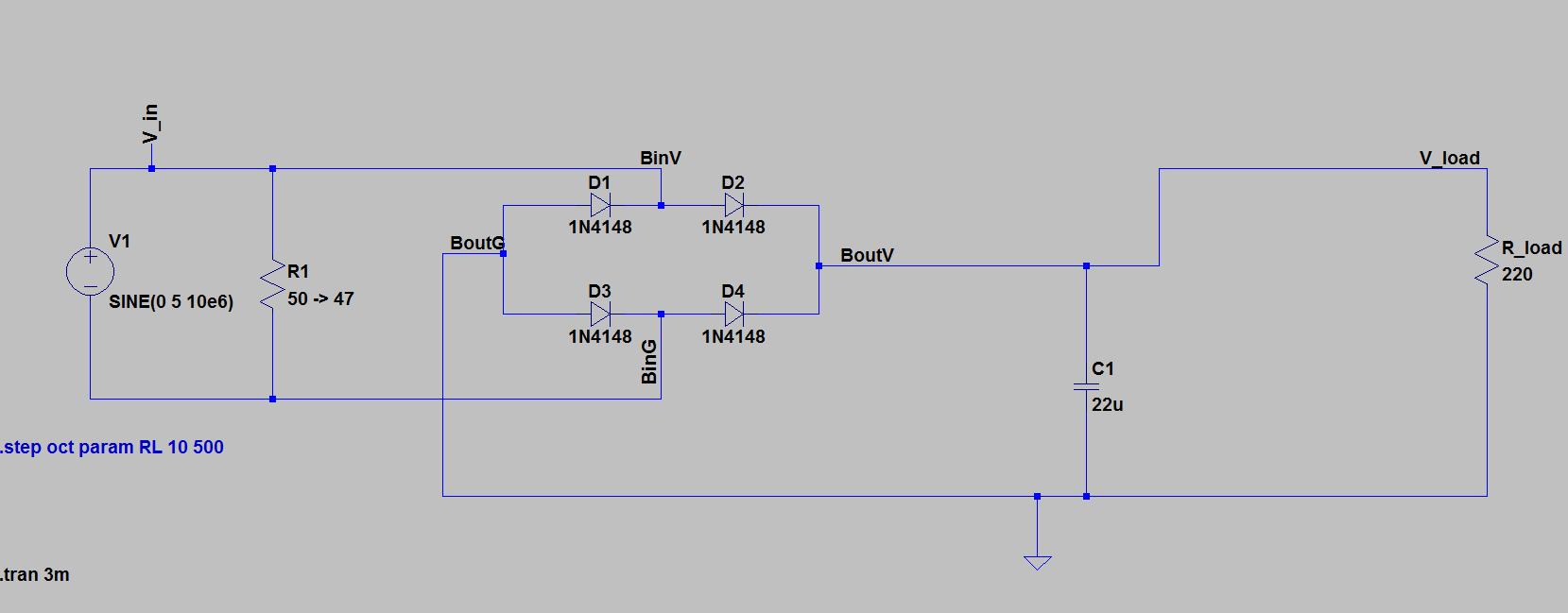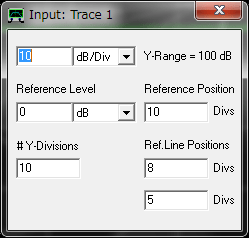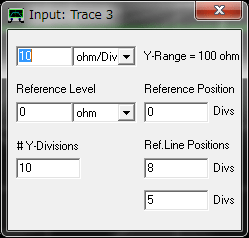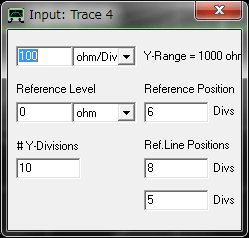I built a rectifier circuit for WPT project.  This rectifier is used at receiver to convert AC from the coil to DC. Here are the pictures and the circuit diagram.

Please look at the R1 in left side. The R1 is need to match the impedance of the system. The value should be 50 Ohm, But I use 47 Ohm instead here because of the simplicity of the circuit.  The rectifier is using the full bridge diodes and the LED is terminated to R_load serially.First, We need to setup the measurement program of the VNWA.

At the display setting (keyboard “d”) : Let the traces are enable, Trace 1,2,3,4,6

Trace Setting

 Trace 1 Trace 2 Trace 3 Trace 4 Trace 5 Trace 6 Menu (right side of the color bar) S11 S11 S11 S11 S11 Function dB VSWR realZ imagZ Smith Color setting on color bar Blue Pink Green Brown RedSecond, We set the frequency range. (keyboard “f”)

In this WPT project, the frequency of interest is 14 MHz. Therefore the frequency range is between  1 to 27 MHz. This setting results in the center frequency to be 14 MHz.Third, We set the VNWA sweep setting. (keyboard “s”)

Number of Datapoints = 2000

Measurement Time = 20 sec

Time per data point = 10msAnd we calibrate the VNWA with Short, Open and Load.

Finally, We set the scale of vertical axis of each parameters.  This is convenient when we need to compare the measurement results with different devices.

1.  Trace 1 : S11 dB2. Trace 2: VSWR3. Trace 3: real Z4. Trace 4: imag ZOk. The setting is done. Now I will show you how to measure the impedance of the rectifier and how the impedance will be.This is the measurement results at f= 14 MHz

 Parameter Value Unit S11 dB -27.84 dB VSWR 1.08 Real Z 46.22 Ohm Imag Z 0.96 Ohm S11 -0.04 + j 0.01

The impedance value is Z = 46.22 j 0.96 [Ohm] (=Real Z + j imagZ ) . This is not so bad result for the first trial.

Did you remember that I insert the R1 of 47 [Ohm] in the circuit.  That results in the Real (Z) = 46.22 [Ohm]

Ok, I am glad to check the impedance is not so far from the 50 Ohm.

Reference

Gerfried Palme, “Measurements with the DG8SAQ VNWA 2/3 Vector Network Analyzer”,  Pressel Digitaler Produkitionsdruck, Germany, 2015

##### Similar Posts

このサイトはスパムを低減するために Akismet を使っています。コメントデータの処理方法の詳細はこちらをご覧ください